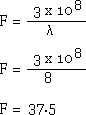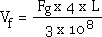# Understanding RF Transmission Lines by Measurement and Calculation page 6

5.1) Velocity of Propagation

The velocity of a signal in free space is the speed of light or 2.997925 x 108 m/s (this is generally accepted to be approximately 3 x 108 m/s). A theoretical loss less coaxial cable working at high frequencies has a velocity of propagation that can be calculated from Equation 5.1.Equation 5.1

Where vp is the velocity of propagation in m/s

C is the distributed capacitance per unit length in pF

L is the distributed inductance per unit length in µH

Equation 5.1 is from TRANSMISSION LINES AND NETWORKS. INTERNATIONAL STUDENTS EDITION by Walter C. Johnson, 1963 by McGraw-Hill Book Co. Singapore ISBN 0-07-Y85348-7, Page 11, and it is shown how this Equation is derived.

The velocity of a signal in a medium is less than that in free space and is dependent on the permeability and permittivity of the materials used as in Equation 5.2.Equation 5.2

Where vp is the velocity of propagation in m/s

c is the speed of light in m/s

μr is the relative permeability

εr is the relative permittivity

In a coaxial cable it is normal practice to use materials that are non-magnetic with μr = 1 and the velocity of propagation then becomes almost completely dependant on the properties of the dielectric.

Equation 5.2 is from Transmission Line Design Handbook by Brian C Wadell, Artech House 1991 page 17 (2.3.9).

5.2) Velocity Factor of a Coaxial Cable

If the dielectric constant of the cable (εr) is known, the approximate velocity factor can be calculated using equation 5.3.Equation 5.3

It is possible to measure the velocity factor of a transmission line using the voltage output of the V/C Detector to detect phase cancellation. If a REFLECTED signal in a coaxial cable is due to an OPEN termination, then at a point a quarter wave length (90°) from that OPEN termination the REFLECTED signal will be 90° + 90° = 180° out of phase with the INCIDENT
signal and a voltage cancellation will result. Although we are measuring voltage, it is the direction of the currents in the voltmeter path of the test equipment that determines the result. Figure 5.0 shows this in more detail and it can be seen that the two very small currents flowing through the voltmeter (IVf and IVr) travel in the same direction, which causes these currents to fundamentally add. However, as the two currents are 180° out of phase in this particular measurement the final result is a cancellation, giving an indicated voltage null. Considering the current direction in the test equipment and the relative signal phases is important for understanding the reality of what happens in a transmission line measurement and will avoid the misunderstandings that have occurred in traditional teaching. It is also necessary to appreciate that both a voltmeter and an ammeter require power to function, albeit very small, and that a voltmeter is essentially a 'power' meter with a high resistance in series, and an ammeter is a 'power' meter with a low (shunt) resistance in parallel. They both require voltage and current to operate!

Figure 5.0The Two Metre Cable is used at a quarter wavelength (λ/4) to determine the velocity factor using this cancellation effect.

The frequency of a signal in free space of wavelength (λ) can be calculated from:-Equation 5.4

Where λ is the wavelength in metres

F is the frequency in MHz

The frequency of a signal in free space for a full wavelength of 8 metres would be:-The test equipment is connected as per Figure 5.1 with the DMM connected to the detected voltage output of the V/C Detector.

Figure 5.1The Signal Generator is set to give an output of +0 dBm at a frequency of 37.5 MHz. This gives an amplifier output of approximately +15 dBm (1.26 Volt rms at 0.025 Amps into 50 Ohms). The OPEN Termination is connected to the output of the Two Metre Cable.

While observing the DMM, the Signal Generator frequency is slowly reduced until a voltage null is reached. The frequency of the Signal Generator and the indicated voltage are recorded. The overall length of the coaxial cable is measured from the red line of the Voltage and Current Detector along the Two Metre Cable to the red line on the OPEN Termination, and the test assembly length is recorded. The Velocity Factor is calculated using Equation 5.5.Equation 5.5

Where Vf is the velocity factor (there are no units)

Fg is the Signal Generator frequency in Hz

L is the length of the cable in metres

The result obtained can be compared with the manufacturers quoted velocity factor.

Note that the voltage null does not reach zero. This is because the REFLECTED signal has a lower voltage than the INCIDENT signal due to the attenuation of the cable. We record this voltage for use in calculations in later measurements. This result also confirms that the V/C Detector is measuring the SUM voltage of the INCIDENT and REFLECTED sine waves.

1  2  3  4  5  6  7  8  9   >  Pages

Transmission Line Index

Technical Article Index